# AP Chemistry : Atomic Structure and Properties

## Example Questions

### Example Question #10 : Atomic Structure And Electron Configuration

A ground state monovalent cation has the following electron configuration: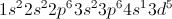What is the cation?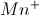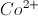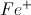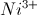Explanation:

Since this is a monovalent cation, the non-ionic electron configuration will be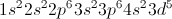(note that the electron was removed from the s shell and not the d shell, as this is a transition metal). This electron configuration corresponds to iron, so the cation must be.

### Example Question #811 : Ap Chemistry

What is the electron configuration for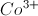?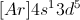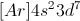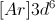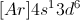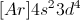Explanation:

First, we will write down the electronic configuration for the ground state of Cobalt:Remember that a half-filled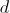orbital is very stable, and when it is possible for an ion to have a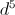electron configuration it will. In order to attain this, two electrons will be removed from theorbitals and one will be removed from the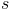orbital, yielding:### Example Question #812 : Ap Chemistry

Below are the data for ionization energies of three elements X, Y, and Z. These elements are in the third period of the periodic table. The first four ionization energies for elements X, Y, and Z are given below in values of kJ/mol.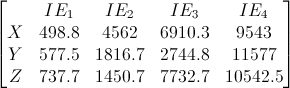Figure 1: Ionization energies in kJ/mol for selected elements.

What is the electron configuration for element X?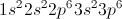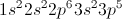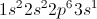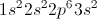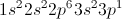Explanation:

We discover that element X is sodium, due to the masive jump after the first ionization energy. Sodium has filled 1s and 2s orbitals, and a half filled 3s orbital. We know that sodium will have the base configuation of [Ne], with one additional electron in the third shell, due to its position in the third period. This leads us to our answer of 1s22s22p63s1.

### Example Question #813 : Ap Chemistry

How many unpaired electrons occupy the 3p orbitals of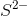?There are no electrons occupying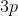orbitalsExplanation:

Atomic sulfur has 16 electrons, yielding 3p orbitals with 2, 1, and 1 electrons as seen below: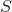orbital diagram:

1s22s23s23p4

We consider the 3p subshell independently as the lower subshells are filled completely. There are three 3p orbitals, each of which may hold two electrons. Hund's rule tells us that each orbital of a given subshell must be singly filled before any orbital in the subshell is doubly filled.

Following this rule, each of the three orbitals are given one of the four remaining electrons, and one orbital is given a second. The 3p subshell has two orbitals occupied by unpaired electrons in atomic sulfur.

However,has two additional electrons. As a result, the 3p orbitals are filled completely and the correct answer is 0 unpaired electrons.orbital diagram:

1s22s23s23p6

Note: this is identical to the electron configuration of Argon.

### Example Question #814 : Ap Chemistry

What is the shorthand electronic configuration for phosphorus?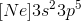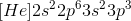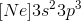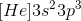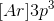Explanation:

In order to write the shorthand electronic configuration correctly, choose the last noble gas before the element of interest. The last noble gas before phosphorus is neon. Put the atomic symbol for neon in brackets and continue assigning electron configurations until phosphorus. After neon, we are in the third row, so the and p orbitals are in the third energy level. Notice that phosphorus has 5 valence electrons since it is in group V. Also notice that in the electron configuration, there are 5 electrons in the highest electron energy level (3).

### Example Question #815 : Ap Chemistry

What is the electron configuration for carbon?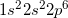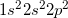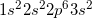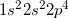Explanation:

Carbon is on the second period and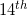group of the periodic table. Each time we jump from left to right, we add an electron. Period 1 corresponds to 1s, period 2 corresponds to 2s, 2p and so forth. The first energy shell can hold a maximum of two electrons, and each subsequent shell can hold eight.

### Example Question #816 : Ap Chemistry

What is the electron configuration for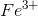?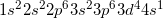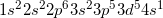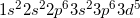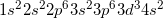Explanation:is on the third period and eighth group of the periodic table. In this problem, you have to consider the ionic state of. This means that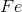is deficient ofelectrons. The complete electron configuration ofat ground state is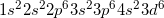. Notice that the electrons are subtracted from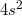first. When electrons are removed from an atom, they are removed from the outermost shell of the atom. In this case, the outermost shell would be from the 4s orbital. However, there are only 2 electrons in this orbital so the remaining one electron would be taken from next highest energy orbital which would be the 3d orbital.

### Example Question #817 : Ap Chemistry

The elements that have an electronic structure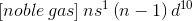:

are Zn, Cd, Hg

are Cu, Ag, Au and can have a stable oxidation state of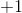belong to group 12 of the periodic table

are not transition metals

are Cu, Ag, Au and can have a stable oxidation state ofExplanation:

Element in the group 11 (Cu, Ag and Au) prefer to have a closed shell configuration for the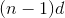orbitals because is energetically more stable. This lives only one electron in the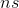orbital. Theoxidation state is easy to achieve because the formed cation exhibits a closed shell configuration for his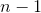level. The last is known as the 18 electrons rule.

### Example Question #818 : Ap Chemistry

Which of the following gives the electron configuration of the chromium atom?

[Ar]4s13d5

[Ar]4s14d5

[Ar]4s23d4

[Ar]3d5

[Ar]4s13d5

Explanation:

For transition metals with six valence electrons, the d orbital is half-filled and s orbital electron is taken to achieve that. The half-filled orbital in the form [Ar]4s13d5, is more stable than in the form [Ar]4s23d4.

[Ar]4s14d5 is wrong because period 4 transition metals need to fill the 3d orbital before the 4d orbital, under the Aufbau principle.

[Ar]3d5 is wrong because it has an incorrect number of electrons.

### Example Question #821 : Ap Chemistry

Which of the following gives the correct electron configuration of chlorine?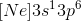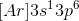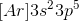Explanation:

This question is asking for the electron configuration of chlorine. From the periodic table, we can see that chlorine is found in group seven as one of the halogens. Furthermore, it is found on the third row of the table.

Recall that as one progresses, one element at a time, through the periodic table, one proton and one electron are added each time. For chlorine, because it is found in the seventh column of the table, we know that it has seven valence electrons.

Moreover, when writing the electron configuration of the elements, it's often useful to use a shorthand to make things easier. This shorthand consists of using the last noble gas to indicate the electron configuration of all the inner shell electrons. In the case of chlorine, all of its inner shell electrons are equivalent to neon.

So instead of writing out all of the electrons in each of their shells and subshells (which can get very long), it's useful to put the last noble gas in brackets to indicate the inner shell electrons. After the bracket, the valence shell electrons are shown.

Also, it's important to remember that shells of a lower energy level are filled before higher level energy shells. Because p orbitals are always higher in energy than s orbitals, the s orbitals of the chlorine atom will fill first, followed by the p orbitals. Thus, the correct electron configuration for chlorine can be shown as.